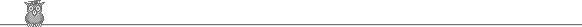### 41st IMO 2000 shortlistProblem A4

The function f on the non-negative integers takes non-negative integer values and satisfies f(4n) = f(2n) + f(n), f(4n+2) = f(4n) + 1, f(2n+1) = f(2n) + 1 for all n. Show that the number of non-negative integers n such that f(4n) = f(3n) and n < 2m is f(2m+1).

Solution

f(4.0) = f(2.0) + f(0), so f(0) = 0. Hence f(1) = f(2.0+1) = f(0) + 1 = 1, f(2) = f(4.0+2) = f(0) + 1 = 1. Similarly, f(3) = f(2.1+1) = f(2) + 1 = 2. Calculating a few more terms, in a similar way, we get:

```n	0   1   2   3   4   5   6   7   8   9  10  11  12  13  14  15  16  17  18  19  20  21
f(n)	0   1   1   2   2   3   3   4   3   4   4   5   5   6   6   7   5   6   6   7   7   8
```
The pattern is not obvious.

However, we may notice f(4n) = f(2n) + f(n). That implies that f(2k+2) = f(2k+1) + f(2k). So if we put f(2k) = fk, then fk is just the kth Fibonacci number. A little further study may then suggest that each f(n) is a sum of Fibonnacci numbers. In fact, if n = bk...b0 in binary, then f(n) = bkfk+1 + ... + b0f1.

To prove this, we just have to verify the equalities in the question. Suppose n = bk ... b0. Then f(n) + f(2n) = (bkfk+1 + ... + b0f1) + (bkfk+2 + ... + b0f2) = bkfk+3 ... b0f3 = f(4n), which establishes the first equality. The second and third are obvious.

We now claim that f(3n) = f(4n) iff no two 1s appear in adjacent positions in the binary expansion of n.

Granting the claim, it remains to show that there are fm+2 integers < 2m with no adjacent 1s. We prove this by induction on m. For m = 1, we have 0 and 1, and f3 = 2. For m = 2, the integers are 0, 1, 3 = 102, and f4 = 3. Suppose it is true for integers < m. Take n < 2m. Let the binary expansion of n be bm-1 ... b0. Then n < 2m-1 iff bm-1 = 0. In this case there are fm+1 possible numbers (by induction). If 2m-1 ≤ n < 2m, then bm-1 = 1, so bm-2 = 0. There are then fm possibilities for the remaining digits (by induction). So in total there are fm + fm+1 = fm+2 possible numbers.

It remains to prove the claim. Suppose there are no adjacent 1s. Then 3n = 2n + n, and there are no carries when this is computed in binary. So if f(n) = bkfk+1 + ... + b0f1, then f(3n) = bk(fk+1 + fk+2) + ... + b0(f1 + f2) = bkfk+3 + ... + b0f3 = f(4n).

The reverse implication is harder. Let Sm be the statement that for 0 < n < 2m we have f(3n) ≤ f(4n) with equality only if the 1s are isolated. For m = 1 we must have n = 1. We have f(3) = f(4) = 2, so S1 is true. So assume Sm is true. Let n = 2m + k with 0 ≤ k < 2m. Then f(4n) = f(2m+2 + 4k) = fm+3 + f(4k).

There are three cases to consider.

Case 1. 0 < k < 2m/2. Then 3k < 2m, so the binary representations of 3.2m = 2m+1 + 2m and 3k do not overlap. So f(3n) = f(2m+1 + 2m + 3k) = fm+2 + fm+1 + f(3k) = fm+3 + f(3k) ≤ fm+3 + f(4k) (by induction) = f(4n) and we have equality only if the 1s are isolated (since k < 2m, if the 1s are isolated in k, then the 1s are also isolated in n = 2m + k).

Case 2. 2m/3 < k < 2m+1/3. Then 3k = 2m + h with h < 2m, so f(3k) = fm+1 + f(h). But 3n = 2(m+1 + 2m) + 3k = 2m+2 + h, so f(3n) = fm+3 + f(h) = fm+3 + f(3k) - fm+1 = fm+2 f(3k) ≤ fm+2 + f(4k) (by induction) < fm+3 + f(3k). We cannot have equality in this case.

Case 3. 2m+1/3 < k < 2m. So 3k = 2m+1 + h with h < 2m, so f(3k) = fm+2 + f(h). We have 3n = (2m+1 + 2m) + 2m+1 + h = 2m+2 + 2m + h, so f(3n) = fm+3 + fm+1 + f(h) = fm+3 - fm+2 + fm+1 + f(3k) < fm+3 + f(3k) ≤ fm+3 + f(4k) = f(4n). We cannot have equality in this case. That completes the proof of the claim.